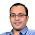### Matrix Puzzle - Math Puzzle

Source: www.puzzletweeter.com

Problem:
Let A,B be 2x2 matrices with integer entries.
Suppose the matrices A,A+B,A+2B,A+3B,A+4B are all invertible and their inverses are also integer matrices.

Then show that A+5B is invertible and it's inverse is an integer matrix.

Update (24 June 2014):
Solution: Posted by Yashoteja Prabhu (Ex-RA at Microsoft Research, IITB CSE 2011 Alumnus) and Sanchit Gupta in comments!

1.first guess; A is identity and B is zero matrix?

1.Given A and B such that... you are not supposed to find A and B :)

2.If P and inv(P) are both integer matrices, then both det(P) and det(inv(P)) are integers. Since det(inv(P)) = 1/det(P), this means det(P) = +1 or -1.

Now det(A+pB) can be written as a quadratic in p, say:
det(A+pB) = a*p^2 + b*p + c
Then these 5 equations should hold by assumption:
|c| = 1,
|a + b + c| = 1
|4a + 2b + c| = 1
|9a + 3b + c| = 1
|16a + 4b + c| = 1

Theorem
=======
Now a (strictly convex) quadratic can take same value only at 2 distinct domain points. If it takes same value at 3 distinct domain points, this means the quadratic is in fact a constant, i.e., a = b = 0,

Since we have five equations and each equation has only 2 possible values in rhs (+1 or -1), at least 3 equations will have the same value.
Hence invoking the theorem, a=b=0,
which implies |25a + 4b + c| = |c| = 1, which further means:
inv(A+pB) exists and is integral for all values of p including p=5.

Proof of theorem
=================

If there exists 3 distinct points p,q,r such that: ap^2+bp+c = aq^2+bq+c = ar^2+br+c,
then subtracting pairs of equations:
(p-q)(a(p+q)+b) = (q-r)(a(q+r)+b) = 0
since p!=q and q!=r:
a(p+q)+b = a(q+r)+b = 0
since, p!=r, this would mean: a = b = 0

1.Good solution. Thanks

One clarification required:
I see that |det(A+pB)| = 1 and so inverse exists
How did you reach to the conclusion that inverse is integral?

2.Yes it does not immediately follow.
Inverse of [a b;c d] is [d -b;-c a]/(ad-bc)
If a,b,c and d are integers and determinant is +/- 1 => inverse will be integral too

Now for p=5, A+pB is integral, and (as said before) determinant is +/- 1, hence its inverse is integral too (so integrality does not hold for general p)

3.How did you deduce that det(A+pB) can be written as a*p^2 + b*p + c?

4.By following:
det([p q;r s]) = ps-qr

5.One typo in the solution: "which implies |25a + 5b + c| = |c| = 1, which further means:"
No harm caused though :-)

3.It has a simple solution
Since A+2B,A+3B,A+4B are invertible
(A+2B)*C1 = I
(A+3B)*C2 = I
(A+4B)*C3 = I
Add 2 and 3 and subtract 1
(A+5B)(C2+C3-C1) = I
=>A+5B is invertible and since Ci are integer matrices => A+5B has integer inverse

1.Thanks. Of course to prove that A+5B is invertible, you have to prove that there exists X such that X*(A+5B) and (A+5B)*X are both integer matrices. Following your argument, the other part of the proof can also be done similarly. Thanks

2.Possibly silly question:
Adding 2 and 3 i get
A(C1 + C2 - C3) + B(2*C1 + 3*C2 - 4*C3) = I
How did you get (A+5B)(C2+C3-C1)?